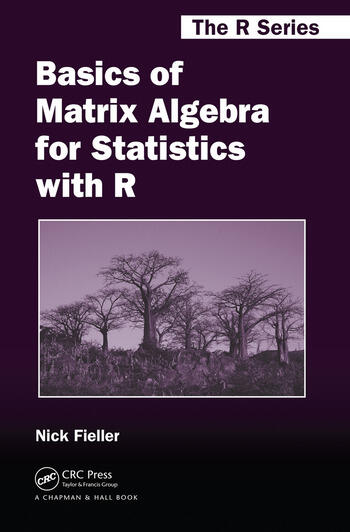# Basics of Matrix Algebra for Statistics with R

## 1st Edition

Nick Fieller

Chapman and Hall/CRC
Published July 6, 2015
Reference - 248 Pages
ISBN 9781498712361 - CAT# K25114
Series: Chapman & Hall/CRC The R Series

For Instructors Request Inspection Copy

was \$64.95

USD\$51.96

SAVE ~\$12.99

FREE Standard Shipping!

## Preview

### Summary

A Thorough Guide to Elementary Matrix Algebra and Implementation in R

Basics of Matrix Algebra for Statistics with R provides a guide to elementary matrix algebra sufficient for undertaking specialized courses, such as multivariate data analysis and linear models. It also covers advanced topics, such as generalized inverses of singular and rectangular matrices and manipulation of partitioned matrices, for those who want to delve deeper into the subject.

The book introduces the definition of a matrix and the basic rules of addition, subtraction, multiplication, and inversion. Later topics include determinants, calculation of eigenvectors and eigenvalues, and differentiation of linear and quadratic forms with respect to vectors. The text explores how these concepts arise in statistical techniques, including principal component analysis, canonical correlation analysis, and linear modeling.

In addition to the algebraic manipulation of matrices, the book presents numerical examples that illustrate how to perform calculations by hand and using R. Many theoretical and numerical exercises of varying levels of difficulty aid readers in assessing their knowledge of the material. Outline solutions at the back of the book enable readers to verify the techniques required and obtain numerical answers.

Avoiding vector spaces and other advanced mathematics, this book shows how to manipulate matrices and perform numerical calculations in R. It prepares readers for higher-level and specialized studies in statistics.

#### Instructors

We provide complimentary e-inspection copies of primary textbooks to instructors considering our books for course adoption.